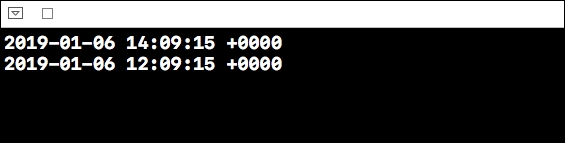# How to subtract 1 hour from current time using Swift?

To subtract hours from a date in swift we need to create a date first. Once that date is created we have to subtract hours from that, though swift does not provide a way to subtract date or time, but it provides us a way to add date or date component in negative value. In this example we’ll see how we can achieve the same.

Let’s create a date first, let it be today,

let today = Date()

Now to modify this date we’ll use the add function with negative value,

let modifiedDate = Calendar.current.date(byAdding: .hour, value: -2, to: today)!

Now to see the difference between both the dates, let’s add print statement for both of these. Our complete code should now look like this.

let today = Date()
print(today)
let modifiedDate = Calendar.current.date(byAdding: .hour, value: -2, to: today)!
print(modifiedDate)

When we run the above code on a playground simulator we get the following result, which shows exactly a difference of two hours in both the date, and the modified date.